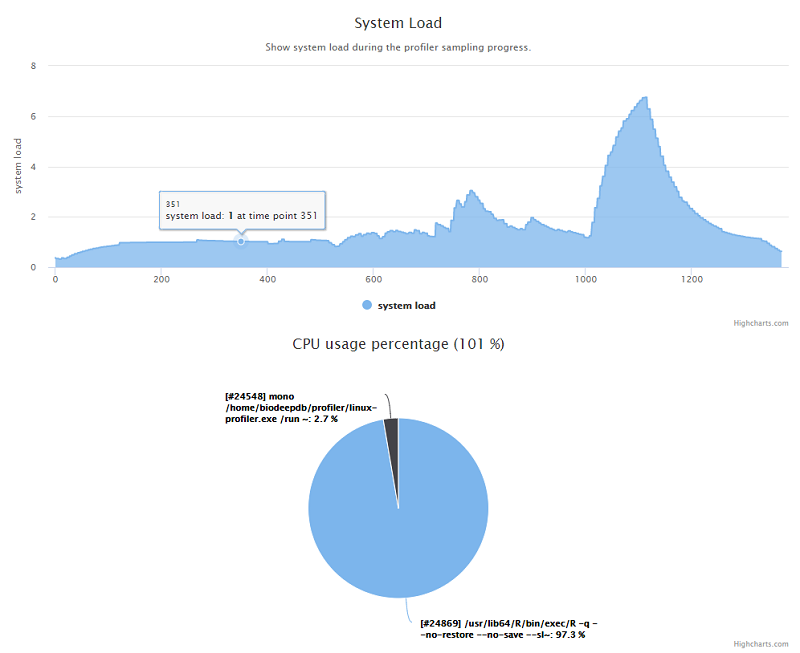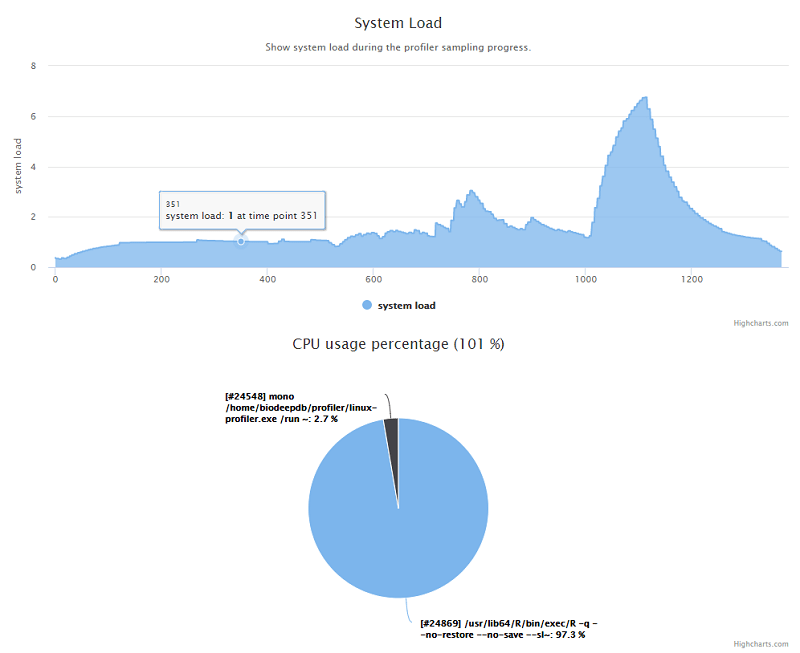## 性能计数器Public Class Snapshot

Public Property uptime As uptime
Public Property timestamp As Double
Public Property mpstat As mpstat()
Public Property free As free
Public Property iostat As iostat
Public Property ps As ps()

Public Function FindAllCPUUsage() As Double
Return mpstat _
.Where(Function(a) a.CPU <> "all") _
.Sum(Function(cpu)
Return cpu.gnice +
cpu.guest +
cpu.iowait +
cpu.irq +
cpu.nice +
cpu.soft +
cpu.steal +
cpu.sys +
cpu.usr
End Function)
End Function

Public Overrides Function ToString() As String
Return uptime.ToString & ", top: " & ps _
.OrderByDescending(Function(a) a.CPU) _
.First _
.COMMAND
End Function
End Class

### 1. CPU利用率### 3. 硬盘I/O<Extension>
Private Function IoSpeed(time2 As Snapshot, time1 As Snapshot, isRead As Boolean) As Double
Dim t2 = DateTimeHelper.FromUnixTimeStamp(time2.timestamp)
Dim t1 = DateTimeHelper.FromUnixTimeStamp(time1.timestamp)
Dim dt As Double = (t2 - t1).TotalSeconds
Dim data2, data1 As Double

data2 = Aggregate dev As iodev In time2.iostat.devices Into Sum(dev.kB_read)
data1 = Aggregate dev As iodev In time1.iostat.devices Into Sum(dev.kB_read)
Else
data2 = Aggregate dev As iodev In time2.iostat.devices Into Sum(dev.kB_wrtn)
data1 = Aggregate dev As iodev In time1.iostat.devices Into Sum(dev.kB_wrtn)
End If

Return (data2 - data1) / dt
End Function

### 4. 系统负载## 性能报告

### 存储数据的JS文件• data/cpuinfo.js 存储CPU的硬件信息
• data/dmidecode.js 存储系统的所有硬件信息
• data/overviews.js 存储系统资源利用率的总览信息
• data/ps.js 存储了每一个快照时间点上所有正在执行的进程列表
• data/system_load.js 存储系统负载的信息

<Extension>
Private Function dataJs(Of T)(data As T, name As String) As String
Return \$"
/**
* @data {GetType(T).FullName}
* @assembly {GetType(T).Assembly.FullName}
* @dll {GetType(T).Assembly.Location.FileName}
*/
function {name}() {{
return {data.GetJson(knownTypes:={GetType(String), GetType(Double())})};
}}"
End Function

## 工具的系统兼容性

Latest posts by 谢桂纲 (see all)